### Home > PC > Chapter 1 > Lesson 1.2.1 > Problem1-69

1-69.
1. Write each expression below with the smallest possible integer base. (Example: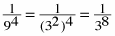= 3−8) (Part (c) may be tricky.) Homework Help ✎

1.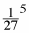2.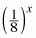3. 16x ·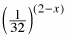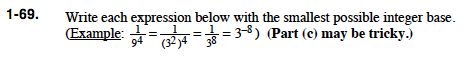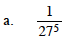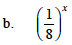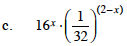Convert the 16 and the 32 to the smallest integer base. Use the Laws of Exponents to combine.

$2x^{9x-10}$